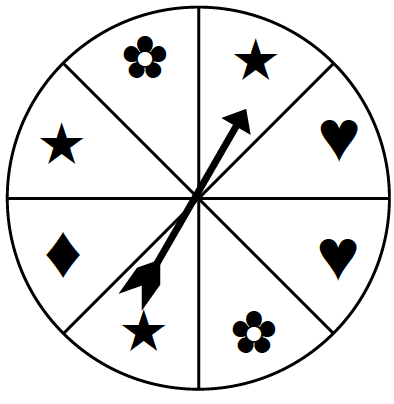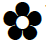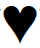### Home > ACC6 > Chapter 2 Unit 10 > Lesson CC2: 2.1.2 > Problem2-28

2-28.Lila is making a spinner game for her cousins to play. She has divided it into $8$ equal sections and has labeled each section with a symbol. When the spinner lands on a flower (), her cousins will win a prize. Create a spinner to explore your ideas!

1. What is P($\bigstar$)? Express your answer as a fraction, as a decimal, and as a percent.

Find the probability of an event by writing a fraction where the number of succesful events is the numerator and the number of possible events is the denominator.

The number of succesful events is $3$, the number of sections in the spinner with a star.

The number of possible events is $8$, the number of sections in the spinner.

$\frac{3}{8},\,0.375$

2. What is the probability of not getting $\blacklozenge$? Write your answer as a fraction, as a decimal, and as a percent.

How many sections of the spinner do not have a diamond shape?

3. What is P()? Write your answer as a fraction, as a decimal, and as a percent.

See the steps for part (a).

4. If Lila’s cousins spin $100$ times, about how many times would you expect them to spin a heart ()?

$25$ times